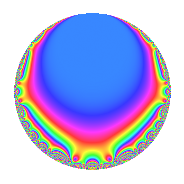Properties

 Label 6014.2.a.cLevel 6014 Weight 2 Character orbit 6014.a Self dual Yes Analytic conductor 48.022 Analytic rank 0 Dimension 2 CM No Inner twists 1

Related objects

Newspace parameters

 Level: $$N$$ = $$6014 = 2 \cdot 31 \cdot 97$$ Weight: $$k$$ = $$2$$ Character orbit: $$[\chi]$$ = 6014.a (trivial)

Newform invariants

 Self dual: Yes Analytic conductor: $$48.0220317756$$ Analytic rank: $$0$$ Dimension: $$2$$ Coefficient field: $$\Q(\sqrt{2})$$ Coefficient ring: $$\Z[a_1, \ldots, a_{5}]$$ Coefficient ring index: $$1$$ Fricke sign: $$-1$$ Sato-Tate group: $\mathrm{SU}(2)$

$q$-expansion

Coefficients of the $$q$$-expansion are expressed in terms of $$\beta = \sqrt{2}$$. We also show the integral $$q$$-expansion of the trace form.

 $$f(q)$$ $$=$$ $$q$$ $$- q^{2}$$ $$+ 2 \beta q^{3}$$ $$+ q^{4}$$ $$+ ( 2 + \beta ) q^{5}$$ $$-2 \beta q^{6}$$ $$+ ( -2 + \beta ) q^{7}$$ $$- q^{8}$$ $$+ 5 q^{9}$$ $$+O(q^{10})$$ $$q$$ $$- q^{2}$$ $$+ 2 \beta q^{3}$$ $$+ q^{4}$$ $$+ ( 2 + \beta ) q^{5}$$ $$-2 \beta q^{6}$$ $$+ ( -2 + \beta ) q^{7}$$ $$- q^{8}$$ $$+ 5 q^{9}$$ $$+ ( -2 - \beta ) q^{10}$$ $$+ 4 q^{11}$$ $$+ 2 \beta q^{12}$$ $$+ ( 2 - \beta ) q^{14}$$ $$+ ( 4 + 4 \beta ) q^{15}$$ $$+ q^{16}$$ $$-\beta q^{17}$$ $$-5 q^{18}$$ $$-6 q^{19}$$ $$+ ( 2 + \beta ) q^{20}$$ $$+ ( 4 - 4 \beta ) q^{21}$$ $$-4 q^{22}$$ $$+ 4 \beta q^{23}$$ $$-2 \beta q^{24}$$ $$+ ( 1 + 4 \beta ) q^{25}$$ $$+ 4 \beta q^{27}$$ $$+ ( -2 + \beta ) q^{28}$$ $$+ ( 4 - 4 \beta ) q^{29}$$ $$+ ( -4 - 4 \beta ) q^{30}$$ $$- q^{31}$$ $$- q^{32}$$ $$+ 8 \beta q^{33}$$ $$+ \beta q^{34}$$ $$-2 q^{35}$$ $$+ 5 q^{36}$$ $$+ ( -4 - 4 \beta ) q^{37}$$ $$+ 6 q^{38}$$ $$+ ( -2 - \beta ) q^{40}$$ $$+ ( 6 - 2 \beta ) q^{41}$$ $$+ ( -4 + 4 \beta ) q^{42}$$ $$+ 6 \beta q^{43}$$ $$+ 4 q^{44}$$ $$+ ( 10 + 5 \beta ) q^{45}$$ $$-4 \beta q^{46}$$ $$+ 4 q^{47}$$ $$+ 2 \beta q^{48}$$ $$+ ( -1 - 4 \beta ) q^{49}$$ $$+ ( -1 - 4 \beta ) q^{50}$$ $$-4 q^{51}$$ $$+ ( 8 + 2 \beta ) q^{53}$$ $$-4 \beta q^{54}$$ $$+ ( 8 + 4 \beta ) q^{55}$$ $$+ ( 2 - \beta ) q^{56}$$ $$-12 \beta q^{57}$$ $$+ ( -4 + 4 \beta ) q^{58}$$ $$+ ( 10 + 2 \beta ) q^{59}$$ $$+ ( 4 + 4 \beta ) q^{60}$$ $$+ ( -4 - 6 \beta ) q^{61}$$ $$+ q^{62}$$ $$+ ( -10 + 5 \beta ) q^{63}$$ $$+ q^{64}$$ $$-8 \beta q^{66}$$ $$+ ( 2 + 6 \beta ) q^{67}$$ $$-\beta q^{68}$$ $$+ 16 q^{69}$$ $$+ 2 q^{70}$$ $$+ ( 2 - 3 \beta ) q^{71}$$ $$-5 q^{72}$$ $$+ ( 2 + 4 \beta ) q^{73}$$ $$+ ( 4 + 4 \beta ) q^{74}$$ $$+ ( 16 + 2 \beta ) q^{75}$$ $$-6 q^{76}$$ $$+ ( -8 + 4 \beta ) q^{77}$$ $$+ ( -2 + 2 \beta ) q^{79}$$ $$+ ( 2 + \beta ) q^{80}$$ $$+ q^{81}$$ $$+ ( -6 + 2 \beta ) q^{82}$$ $$+ ( -4 + 9 \beta ) q^{83}$$ $$+ ( 4 - 4 \beta ) q^{84}$$ $$+ ( -2 - 2 \beta ) q^{85}$$ $$-6 \beta q^{86}$$ $$+ ( -16 + 8 \beta ) q^{87}$$ $$-4 q^{88}$$ $$+ ( -2 + 6 \beta ) q^{89}$$ $$+ ( -10 - 5 \beta ) q^{90}$$ $$+ 4 \beta q^{92}$$ $$-2 \beta q^{93}$$ $$-4 q^{94}$$ $$+ ( -12 - 6 \beta ) q^{95}$$ $$-2 \beta q^{96}$$ $$+ q^{97}$$ $$+ ( 1 + 4 \beta ) q^{98}$$ $$+ 20 q^{99}$$ $$+O(q^{100})$$ $$\operatorname{Tr}(f)(q)$$ $$=$$ $$2q$$ $$\mathstrut -\mathstrut 2q^{2}$$ $$\mathstrut +\mathstrut 2q^{4}$$ $$\mathstrut +\mathstrut 4q^{5}$$ $$\mathstrut -\mathstrut 4q^{7}$$ $$\mathstrut -\mathstrut 2q^{8}$$ $$\mathstrut +\mathstrut 10q^{9}$$ $$\mathstrut +\mathstrut O(q^{10})$$ $$2q$$ $$\mathstrut -\mathstrut 2q^{2}$$ $$\mathstrut +\mathstrut 2q^{4}$$ $$\mathstrut +\mathstrut 4q^{5}$$ $$\mathstrut -\mathstrut 4q^{7}$$ $$\mathstrut -\mathstrut 2q^{8}$$ $$\mathstrut +\mathstrut 10q^{9}$$ $$\mathstrut -\mathstrut 4q^{10}$$ $$\mathstrut +\mathstrut 8q^{11}$$ $$\mathstrut +\mathstrut 4q^{14}$$ $$\mathstrut +\mathstrut 8q^{15}$$ $$\mathstrut +\mathstrut 2q^{16}$$ $$\mathstrut -\mathstrut 10q^{18}$$ $$\mathstrut -\mathstrut 12q^{19}$$ $$\mathstrut +\mathstrut 4q^{20}$$ $$\mathstrut +\mathstrut 8q^{21}$$ $$\mathstrut -\mathstrut 8q^{22}$$ $$\mathstrut +\mathstrut 2q^{25}$$ $$\mathstrut -\mathstrut 4q^{28}$$ $$\mathstrut +\mathstrut 8q^{29}$$ $$\mathstrut -\mathstrut 8q^{30}$$ $$\mathstrut -\mathstrut 2q^{31}$$ $$\mathstrut -\mathstrut 2q^{32}$$ $$\mathstrut -\mathstrut 4q^{35}$$ $$\mathstrut +\mathstrut 10q^{36}$$ $$\mathstrut -\mathstrut 8q^{37}$$ $$\mathstrut +\mathstrut 12q^{38}$$ $$\mathstrut -\mathstrut 4q^{40}$$ $$\mathstrut +\mathstrut 12q^{41}$$ $$\mathstrut -\mathstrut 8q^{42}$$ $$\mathstrut +\mathstrut 8q^{44}$$ $$\mathstrut +\mathstrut 20q^{45}$$ $$\mathstrut +\mathstrut 8q^{47}$$ $$\mathstrut -\mathstrut 2q^{49}$$ $$\mathstrut -\mathstrut 2q^{50}$$ $$\mathstrut -\mathstrut 8q^{51}$$ $$\mathstrut +\mathstrut 16q^{53}$$ $$\mathstrut +\mathstrut 16q^{55}$$ $$\mathstrut +\mathstrut 4q^{56}$$ $$\mathstrut -\mathstrut 8q^{58}$$ $$\mathstrut +\mathstrut 20q^{59}$$ $$\mathstrut +\mathstrut 8q^{60}$$ $$\mathstrut -\mathstrut 8q^{61}$$ $$\mathstrut +\mathstrut 2q^{62}$$ $$\mathstrut -\mathstrut 20q^{63}$$ $$\mathstrut +\mathstrut 2q^{64}$$ $$\mathstrut +\mathstrut 4q^{67}$$ $$\mathstrut +\mathstrut 32q^{69}$$ $$\mathstrut +\mathstrut 4q^{70}$$ $$\mathstrut +\mathstrut 4q^{71}$$ $$\mathstrut -\mathstrut 10q^{72}$$ $$\mathstrut +\mathstrut 4q^{73}$$ $$\mathstrut +\mathstrut 8q^{74}$$ $$\mathstrut +\mathstrut 32q^{75}$$ $$\mathstrut -\mathstrut 12q^{76}$$ $$\mathstrut -\mathstrut 16q^{77}$$ $$\mathstrut -\mathstrut 4q^{79}$$ $$\mathstrut +\mathstrut 4q^{80}$$ $$\mathstrut +\mathstrut 2q^{81}$$ $$\mathstrut -\mathstrut 12q^{82}$$ $$\mathstrut -\mathstrut 8q^{83}$$ $$\mathstrut +\mathstrut 8q^{84}$$ $$\mathstrut -\mathstrut 4q^{85}$$ $$\mathstrut -\mathstrut 32q^{87}$$ $$\mathstrut -\mathstrut 8q^{88}$$ $$\mathstrut -\mathstrut 4q^{89}$$ $$\mathstrut -\mathstrut 20q^{90}$$ $$\mathstrut -\mathstrut 8q^{94}$$ $$\mathstrut -\mathstrut 24q^{95}$$ $$\mathstrut +\mathstrut 2q^{97}$$ $$\mathstrut +\mathstrut 2q^{98}$$ $$\mathstrut +\mathstrut 40q^{99}$$ $$\mathstrut +\mathstrut O(q^{100})$$

Embeddings

For each embedding $$\iota_m$$ of the coefficient field, the values $$\iota_m(a_n)$$ are shown below.

For more information on an embedded modular form you can click on its label.

Label $$\iota_m(\nu)$$ $$a_{2}$$ $$a_{3}$$ $$a_{4}$$ $$a_{5}$$ $$a_{6}$$ $$a_{7}$$ $$a_{8}$$ $$a_{9}$$ $$a_{10}$$
1.1
 −1.41421 1.41421
−1.00000 −2.82843 1.00000 0.585786 2.82843 −3.41421 −1.00000 5.00000 −0.585786
1.2 −1.00000 2.82843 1.00000 3.41421 −2.82843 −0.585786 −1.00000 5.00000 −3.41421
 $$n$$: e.g. 2-40 or 990-1000 Significant digits: Format: Complex embeddings Normalized embeddings Satake parameters Satake angles

Inner twists

This newform does not admit any (nontrivial) inner twists.

Atkin-Lehner signs

$$p$$ Sign
$$2$$ $$1$$
$$31$$ $$1$$
$$97$$ $$-1$$

Hecke kernels

This newform can be constructed as the kernel of the linear operator $$T_{3}^{2}$$ $$\mathstrut -\mathstrut 8$$ acting on $$S_{2}^{\mathrm{new}}(\Gamma_0(6014))$$.